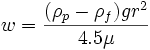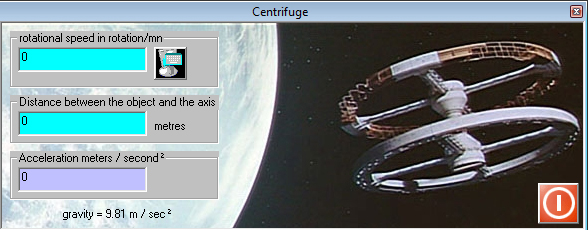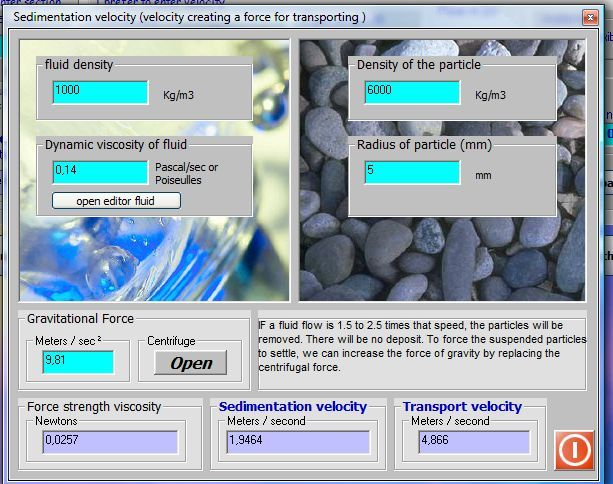Sedimentation velocity of a particle is the minimum speed that a flood must have to carry, rather than drop, particles or sediment.. Sedimentation velocity of a particle is given by Stokes' law:$speed sedimentation$

where w is the sedimentation rate, ρ is the density ( p and f respectively indicate particle and fluid), g is the acceleration due to gravity, r is the radius of the particle and μ is the dynamic viscosity of the fluid.

Low Sedimentation Rate particle means that the particle has a tendency to "float" in the fluid. A high sedimentation rate indicates that the particle "quickly sank to the bottom of fluid". To increase the sedimentation velocity of a particle, to make it sinking in a fluid, in order to separate particles , we can increase the gravity factor "g" of equation above, using a centrifuge.

It is thus possible to adjust the speed of the centrifuge so that the sedimentation velocity causes separation of different particles, of a heterogeneous fluid (comprising particles of various types). (see gravity / centrifuge)If the fluid velocity is greater than the deposition rate (sedimentation) the aggregate continues downstream.. But even at the speed of sedimentation in the pipes, deposits can accumulate, agglomerate and create caps ... There are therefore the sedimentation rate, the transport speed. The conveying speed of avoiding aglomeration the particles is 1.5 to 2.5 times higher than the speed of sedimentation.

The interface of calculating Sedimentation Rate in the Tools MECAFLUXAlert "minimum Sedimentation Rate" can be adjusted in the interface sizing of pipes to detect the maximum diameters, not to exceed, in a project network with fluid countaining particles.

The pipelines transporting particules as vacuuming chips or the rainwater, must be dimensioned according to the conveying speed, 1.5 to 2.5 times greater than the rate of sedimentation in order to prevent particle deposition.detail of the interface sizing, hydraulic or air ducts.